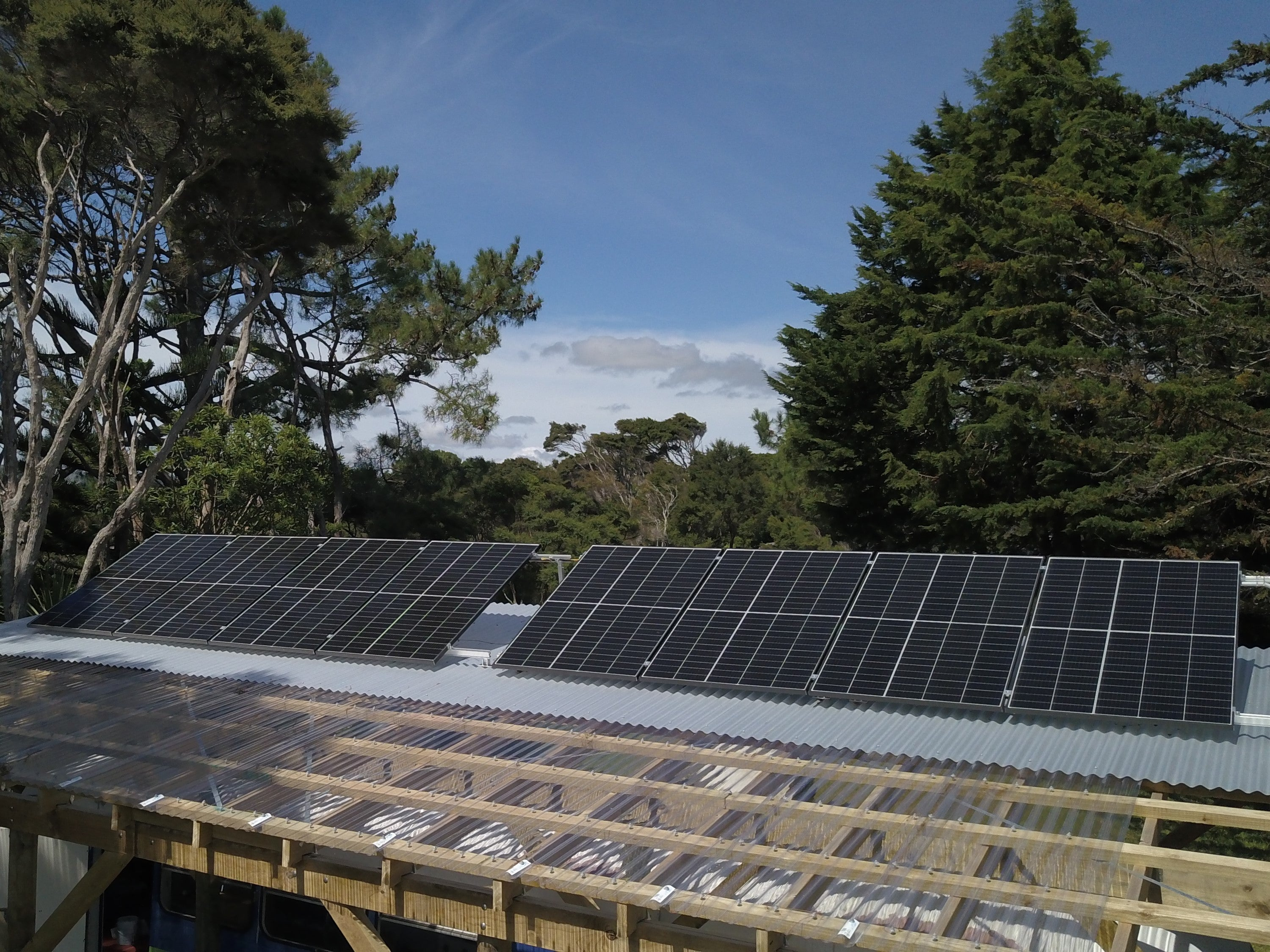# What is a kilowatt hour?

If you’ve started looking into solar, kilowatt hours (kWh) is a term you’ve probably seen – but what does it mean and how do you use this term to find the right solar solution? This article will break it all down for you.

Effects of Shadow on Solar Panels Reading What is a kilowatt hour? 3 minutes

If you’ve started looking into solar, kilowatt hours (kWh) is a term you’ve probably seen – but what does it mean and how do you use this term to find the right solar solution? This article will break it all down for you.

What is a kWh?

Simply, a kilowatt hour (kWh) is a measure of electricity used over time, in hours and thousands of watts. Outside of off-grid solar, you’ll see this measurement on power bills, as you pay per kWh used.

Every appliance will have a wattage rating which tells you how many Watts (W) of power it uses while running – for some it’s very simple, e.g., a 50W lamp will consistently pull 50W while it's on, and for others it’s variable, e.g., a fridge cycles off and on across the day.

Like metres and grams, when the numbers get into the thousands, we usually swap to using 'kilo' (k), so a 2000W oven will also be called a 2kW oven.

If you have the wattage rating, you can multiply it by the hours of usage to calculate the total energy used.

So that 50W lamp would use 25Wh in 30 minutes, or 500W in 10 hours, which is 0.5kWh.

So how do you use that to work out how much solar you need?

Knowing how much power you intend to use will help you work out how much power you need to generate.

We have an article that gives you the full breakdown of how to calculate your power usage, but in short: you need to work out all the appliances you intend to run, how much power they use, and how long you intend to run them. This allows you to calculate your power usage in kWh for an average 24-hour period.

It's easy for items that have a consistent power draw, but items like a fridge or washing machine use more or less power over the course of their operation, so it's hard to know how much power they use.

With these it's easiest to refer to the number on the Energy Star sticker, which is a yearly usage prediction. For a fridge, this is based on 24 hour running, and other appliances will give a use case (eg warm wash 3x a week). If that's similar to your intended usage, you can divide that number by 365 to get a daily estimate.

The total of these numbers gives you an estimate of how much power you need to generate. Solar power generation is also measured in kWh, so it's simple enough to work backwards from your power usage number to discover how many panels you need. You can read further about how to work that our here, or simply use our load calculator tool.

If you need explanations for other solar power terms, you can read our jargon breakdown here